# sfepy.homogenization.convolutions module¶

class sfepy.homogenization.convolutions.ConvolutionKernel(name, times, kernel, decay=None, exp_coefs=None, exp_decay=None)[source]

The convolution kernel with exponential synchronous decay approximation approximating the original kernel represented by the array,.where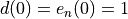,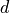is the synchronous decay andits exponential approximation,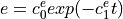.

diff_dt(use_exp=False)[source]

The derivative of the kernel w.r.t. time.

get_exp()[source]

Get the exponential synchronous decay kernel approximation.

get_full()[source]

Get the original (full) kernel.

int_dt(use_exp=False)[source]

The integral of the kernel in time.

sfepy.homogenization.convolutions.approximate_exponential(x, y)[source]

Approximateby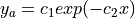.

Initial guess is given by assuming y has already the required exponential form.

sfepy.homogenization.convolutions.compute_mean_decay(coef)[source]

Compute mean decay approximation of a non-scalar fading memory coefficient.

sfepy.homogenization.convolutions.eval_exponential(coefs, x)[source]
sfepy.homogenization.convolutions.fit_exponential(x, y, return_coefs=False)[source]

Evaluateafter approximating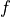by an exponential.